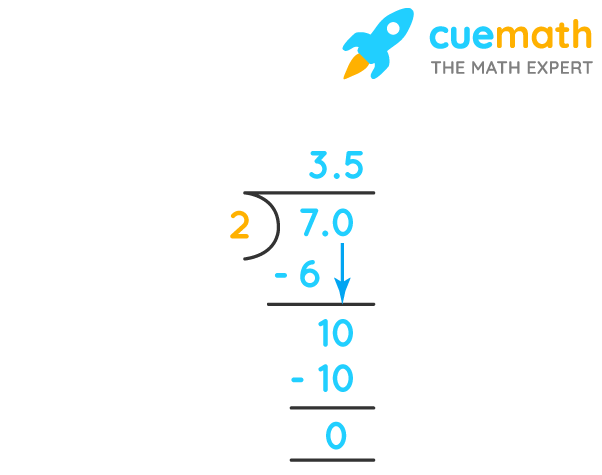# 3½ As A Decimal

## Question: What is 3½ as a decimal?

Both fractions and decimals are just two ways to represent the same numbers.

## Answer: 3½ as a decimal is equal to 3.5.

Let's convert the given mixed fraction to a decimal number.

## Explanation:

3½ can be expressed as 3 + 1/2 = 7/2

To convert any fraction to decimal form, we just need to divide its numerator by denominator.